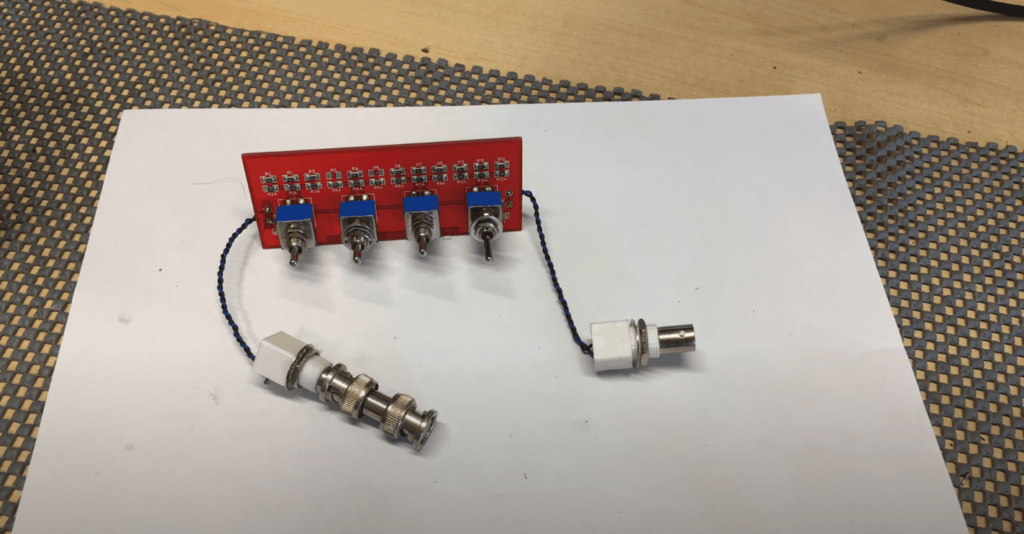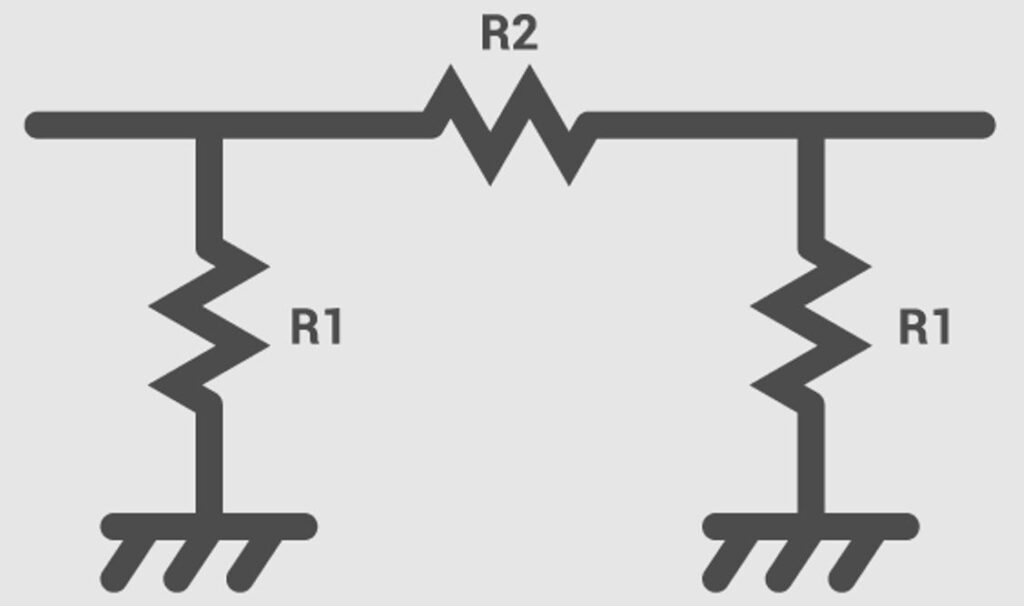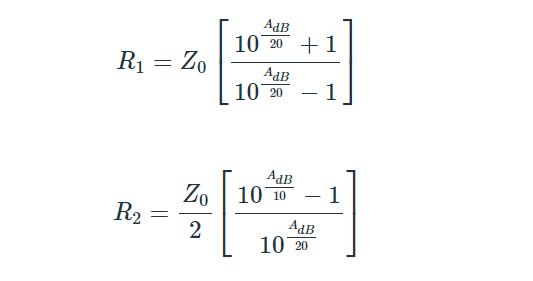# Pi Attenuator Calculator: Online Calculation and Formula

Welcome to the Pi Attenuator Online Calculator! This tool is designed to help you calculate the values of the resistors R1 and R2 to be used for a Pi Attenuator, given the required attenuation in decibels (dB) and the impedance in ohms.

A Pi Attenuator is a passive network commonly used in electronic circuits to reduce the power of a signal without significantly distorting its waveform. The Pi Attenuator consists of three resistors connected in a pi-shaped (Π-shaped) configuration.The values of the resistors R1 and R2 are critical to the performance of the Pi Attenuator, and this calculator makes it easy to determine the optimal values for your circuit. Simply enter the required attenuation and impedance, and the calculator will provide you with the values of R1 and R2. We hope you find this tool helpful for your electronic circuit design needs.

Contents

## Pi Attenuator Calculator for Excel

Formula for R1:

```= B2 * ((10^(A2/20)) + 1) / ((10^(A2/20)) - 1)
```

This formula uses cell A2 to represent the desired attenuation in decibels (dB) and cell B2 to represent the characteristic impedance of the system (Z0). It calculates the value of resistor R1 for the Pi Attenuator.

Formula for R2:

```= (B2^2) / (10^(A2/20)) / ((10^(A2/20)) - 1)
```

This formula also uses cell A2 to represent the desired attenuation in decibels (dB) and cell B2 to represent the characteristic impedance of the system (Z0). It calculates the value of resistor R2 for the Pi Attenuator.

## Pi Attenuator Equations

The Pi Attenuator consists of three resistors connected in a specific configuration, and the values of the resistors can be calculated using the following equations:These equations are used to determine the optimal values of the resistors in a Pi Attenuator circuit.

## FAQ

### What does PI attenuator do?

In terms of application, a PI attenuator is used to match the impedance of two circuits while providing a specified level of attenuation. It is commonly used in RF and audio circuits to provide attenuation to signals to avoid overloading of subsequent stages or reduce the signal level to a more appropriate level for processing or output. A line-level attenuator typically has lower power handling and controls preamp-level signals, whereas a power attenuator is used between the power amplifier and the speaker to manage high-power signals.

### What is the difference between T and PI attenuators?

Both T and Pi attenuators are passive electronic circuits used to reduce the signal level. The difference between them lies in their circuit topology. In a T attenuator, the two series resistors are connected to the input and output of the circuit, with a shunt resistor placed between them. This creates a T-shaped network. In contrast, a Pi attenuator has two parallel resistors connected in series between the input and output, forming a Pi-shaped network.

The key difference between the two types of attenuators is the placement of the shunt resistor or parallel resistors. In a T attenuator, the shunt resistor is used to attenuate the signal, whereas in a Pi attenuator, parallel resistors are used to provide the attenuation. As a result, the resistance values in a T attenuator can be added together, while in a Pi attenuator, the resistors in parallel require a more complex calculation.

### Where are attenuators mainly used?

Attenuators are used in various applications where signal power or voltage reduction is required. Some common uses of attenuators include audio systems, RF (radio frequency) communication systems, microwave systems, and testing and measurement equipment. They can also be used in power amplifiers to reduce power and protect sensitive components such as speakers or headphones. In short, attenuators are used wherever signal levels need to be adjusted and signal attenuation is required.

## Related Video: Attenuator Circuits

The Pi Attenuator Calculator is a useful tool for determining the resistor values needed for a Pi Attenuator based on the desired attenuation and impedance. By using the provided equations and entering the appropriate values, users can easily calculate the resistor values required for their specific application. This calculator can be especially helpful for those working with audio or RF circuits, where attenuation is often needed to achieve the desired signal level. Overall, the Pi Attenuator Calculator is a valuable resource for anyone working with attenuators in their electronic designs.

Scroll to Top